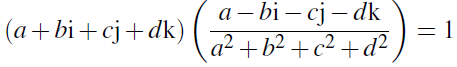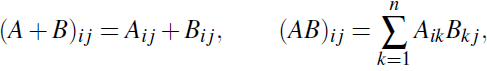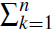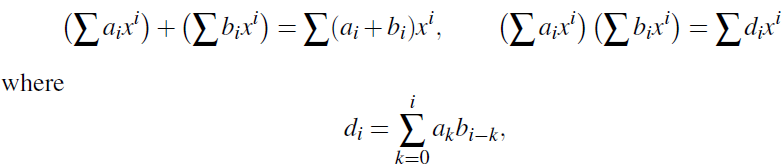Definition of a ring: A ring has two operations: the first is called addition and is denoted by (with infix notation); the second is called multiplication, and is usually denoted by juxtaposition (but sometimes by · with infix notation). In order to be a ring, the structure must satisfy certain rules called axioms. We group these into three classes. The name of the ring is R. We define a ring to be a set R with two binary operations satisfying the following axioms:

(A0) (Closure law) For any a,b 2 R, we have a b 2 R.
(A1) (Associative law) For any a,b,c 2 R, we have (a b) c=a (b c).
(A2) (Identity law) There is an element 0 2 R with the property that a 0 = 0 a = a for all a 2 R. (The element 0 is called the zero element of R.)
(A3) (Inverse law) For any element a 2 R, there is an element b 2 R satisfying a b = b a = 0. (We denote this element b by −a, and call it the additive inverse or negative of a.)

(A4) (Commutative law) For any a,b 2 R, we have a b = b a. Axioms for multiplication:
(M0) (Closure law) For any a,b 2 R, we have ab 2 R.
(M1) (Associative law) For any a,b,c 2 R, we have (ab)c = a(bc). Mixed axiom:
(D) (Distributive laws) For any a,b,c 2 R, we have (a b)c = ac bc and c(a b) = ca cb.

Remarks

1. The closure laws (A0) and (M0) are not strictly necessary. If is a binary operation, then it is a function from R×R to R, and so certainly a b is an element of R for all a,b 2 R. We keep these laws in our list as a reminder.

2. The zero element 0 defined by (A2) and the negative −a defined by (A3) are not claimed to be unique by the axioms. We will see later on that there is only one zero element in a ring, and that each element has only one negative. Axioms (M0) and (M1) parallel (A0) and (A1). Notice that we do not require multiplicative analogues of the other additive axioms. But there will obviously be some rings in which they hold. We state them here for reference.

Further multiplicative properties
(M2) (Identity law) There is an element 1 2 R such that a1 = 1a = a for all a 2 R. (The element 1 is called the identity element of R.)
(M3) (Inverse law) For any a 2 R, if a 6=0, then there exists an element b 2 R such that ab = ba = 1. (We denote this element b by a−1, and call it the multiplicative inverse of a.)
(M4) (Commutative law) For all a,b 2 R, we have ab = ba.

A ring which satisfies (M2) is called a ring with identity; a ring which satisfies (M2) and (M3) is called a division ring; and a ring which satisfies (M4) is called a commutative ring. (Note that the term “commutative ring” refers to the fact that the multiplication is commutative; the addition in a ring is always commutative!) A ring which satisfies all three further properties (that is, a commutative division ring) is called a field.

Examples of rings
1. The integers: The most important example of a ring is the set Z of integers, with the usual addition and multiplication. The various properties should be familiar to you; we will simply accept that they hold. Z is a commutative ring with identity. It is not a division ring because there is no integer b satisfying 2b = 1. This ring will be our prototype for several things in the course.
Note that the set N of natural numbers, or non-negative integers, is not a ring, since it fails the inverse law for addition. (There is no non-negative integer b such that 2 b = 0.)
2. Other number systems: Several other familiar number systems, namely the rational numbers Q, the real numbers R, and the complex numbers C, are fields. Again, these properties are assumed to be familiar to you.
3. The quaternions: There do exist division rings in which the multiplication is not commutative, that is, which are not fields, but they are not so easy to find. The simplest example is the ring of quaternions, discovered by Hamilton in 1843.
Instead of adding just one element i to the real numbers, Hamilton added three. That is, a quaternion is an object of the form a bi cj dk, where
i2 = j2 = k2 = −1, ij = −ji = k, jk = −kj = i, ki = −ik = j.
It can be shown that all the axioms (A0)–(A4), (M0)–(M3) and (D) are satisfied.

For example, if a,b,c,d are not all zero, then we haveThe ring of quaternions is denoted by H, to commemorate Hamilton.

4. Matrix rings: The formulae for addition and multiplication of n×n matrices, namelyjust depend on the fact that we can add and multiply the entries. In principle these can be extended to any system in which addition and multiplication are possible. However, there is a problem with multiplication, because of the, which tells us to add up n terms. In general we can only add two things at a time, since addition is a binary operation, so we have to make the convention that, for example, a b c means (a b) c, a b c d means (a b c) d, and so on. We will return to this point in the next subsection. Now we have the following result:

5. Polynomial rings: In much the same way, the usual rules for addition of polynomials,can be extended to polynomials with coefficients in any algebraic structure in which addition and multiplication are defined. As for matrices, we have to be able to add an arbitrary number of terms to make sense of the definition of multiplication. We have the result: